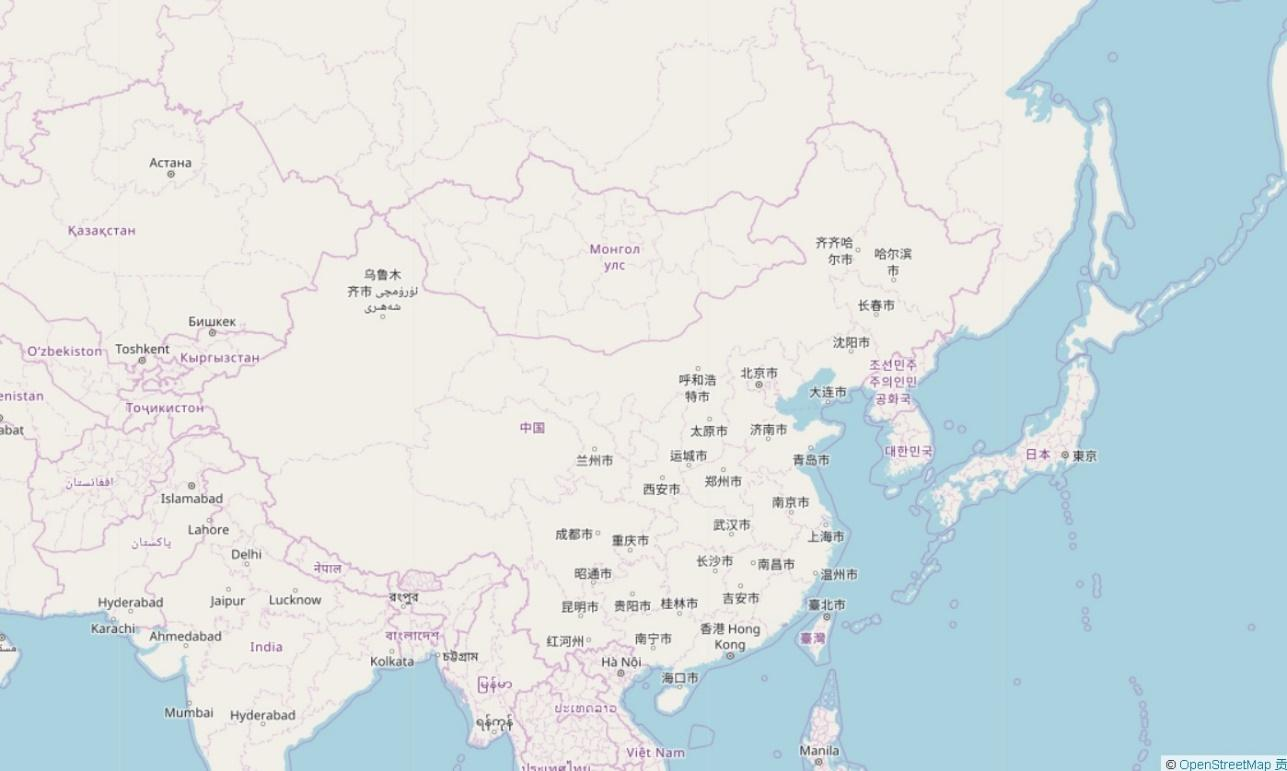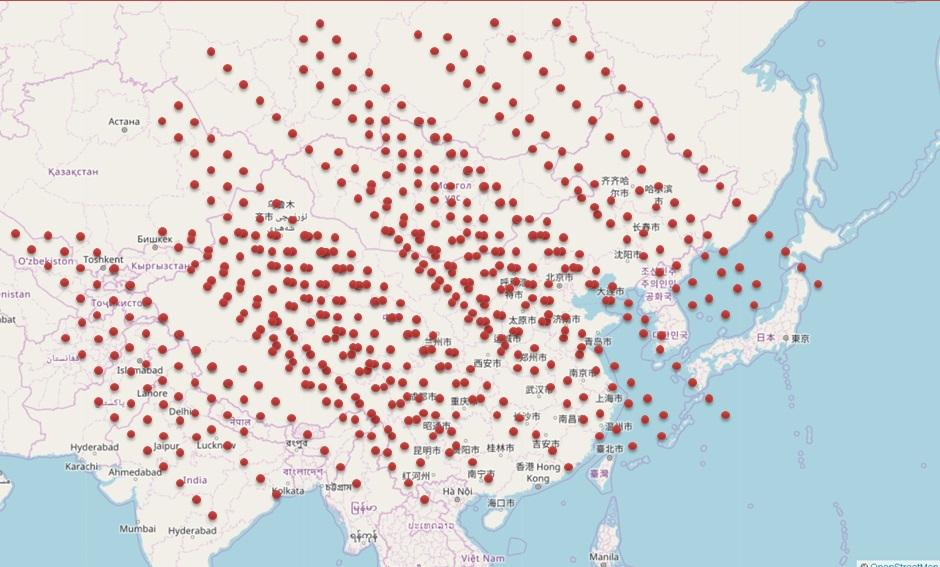# Community

Blog Events Webinars Tutorials Forum
×
Community Blog PostgreSQL: How to Determine the Positions of Overlapping Points and Planes in PostGIS

# PostgreSQL: How to Determine the Positions of Overlapping Points and Planes in PostGIS

In this article, the author discusses overlapping points and planes and determining their actual positions from a visual perspective in PostGIS.

By digoal

## Background

In new retail and express delivery industries, there is a large amount of point data — such as the locations of packages, couriers, and warehouses — and planar data — such as the location of residential areas, business districts, and office buildings.

How to determine a package’s location during delivery? How to connect the location information with each package?

It would be simple if you think about it from a visual perspective.

Here is a map that you can divide into multiple planes (for example, communities).There are many points on this map.From the preceding map, you can observe in which community a point falls in.

In a database, you can store this as two data sets: points and planes. The output is a combination of the point and plane data.

How to obtain the output efficiently?

## DEMO

Often, users query package locations of multiple orders simultaneously. Therefore, determining which planes the queried packages’ points fall in is a typical request that requires a point and plane data combination.

The following example involves 250,000 planes and shows how to query the planes that contain packages from multiple orders.

1) Generate static plane data, for example, data of communities, business districts, buildings, and warehouses.

``````postgres=# create table t2(id int, pos box);
CREATE TABLE

-- 在(0,0)到(500,500)的平面上，划分成251001个正方形的小面。

postgres=# do language plpgsql \$\$
declare
x int;
y int;
begin
for x in 0..500 loop
for y in 0..500 loop
insert into t2 values (x+y, box(point(x,y),point(x+1,y+1)));
end loop;
end loop;
end;
\$\$;
DO

postgres=# select count(*) from t2;
count
--------
251001
(1 row)  ``````

Run the following command to create a spatial index.

``postgres=# create index idx_t2 on t2 using gist(pos);``

2) Generate point data.

``````postgres=# create table t1(id int, pos point);
CREATE TABLE
-- 在(0,0),(500,500)的平面上，生成10000个随机的点

postgres=# insert into t1 select id, point(random()*500, random()*500) from generate_series(1,10000) t(id);
INSERT 0 10000  ``````

3) Query the plane to which a point belongs.

``````Method 1: JOIN
``````
``````postgres=# explain analyze select t1.*,t2.* from t1 join t2 on (t2.pos @> box(t1.pos, t1.pos));
QUERY PLAN
------------------------------------------------------------------------------------------------------------------------
Nested Loop  (cost=0.29..73322.20 rows=2510010 width=56) (actual time=0.094..1191.076 rows=10000 loops=1)
->  Seq Scan on t1  (cost=0.00..116.00 rows=10000 width=20) (actual time=0.020..1.047 rows=10000 loops=1)
->  Index Scan using idx_t2 on t2  (cost=0.29..4.81 rows=251 width=36) (actual time=0.039..0.118 rows=1 loops=10000)
Index Cond: (pos @> box(t1.pos, t1.pos))
Planning time: 0.102 ms
Execution time: 1191.619 ms
(6 rows)  ``````

Method 2: SUBQUERY

``````postgres=# explain analyze select t1.*, (select t2 from t2 where t2.pos @> box(t1.pos,t1.pos) limit 1) from t1;
QUERY PLAN
----------------------------------------------------------------------------------------------------------------------------------
Seq Scan on t1  (cost=0.00..13706.74 rows=10000 width=52) (actual time=0.077..427.466 rows=10000 loops=1)
SubPlan 1
->  Limit  (cost=0.29..1.36 rows=1 width=60) (actual time=0.042..0.042 rows=1 loops=10000)
->  Index Scan using idx_t2 on t2  (cost=0.29..269.88 rows=251 width=60) (actual time=0.042..0.042 rows=1 loops=10000)
Index Cond: (pos @> box(t1.pos, t1.pos))
Planning time: 0.080 ms
Execution time: 427.942 ms
(7 rows)  ``````

For 1000 orders, the result returns in about 40 ms.

``````postgres=# explain (analyze,verbose,timing,costs,buffers) select t1.*, (select t2 from t2 where t2.pos @> box(t1.pos,t1.pos) limit 1) from t1 limit 1000;
QUERY PLAN
----------------------------------------------------------------------------------------------------------------------------------------------
Limit  (cost=0.00..1370.67 rows=1000 width=52) (actual time=0.069..39.754 rows=1000 loops=1)
Output: t1.id, t1.pos, ((SubPlan 1))
Buffers: shared hit=3002
->  Seq Scan on public.t1  (cost=0.00..13706.74 rows=10000 width=52) (actual time=0.069..39.658 rows=1000 loops=1)
Output: t1.id, t1.pos, (SubPlan 1)
Buffers: shared hit=3002
SubPlan 1
->  Limit  (cost=0.29..1.36 rows=1 width=60) (actual time=0.039..0.039 rows=1 loops=1000)
Output: t2.*
Buffers: shared hit=3000
->  Index Scan using idx_t2 on public.t2  (cost=0.29..269.88 rows=251 width=60) (actual time=0.039..0.039 rows=1 loops=1000)
Output: t2.*
Index Cond: (t2.pos @> box(t1.pos, t1.pos))
Buffers: shared hit=3000
Planning time: 0.066 ms
Execution time: 39.830 ms
(16 rows)``````

For now, @> doesn't support "hash join", so we recommend subquery.

For the demonstration convenience, a built-in geometry type is used instead of PostGIS.

However, you need to use PostGIS in real cases.

Example:

``select  t1.*, (select t2 from t2 where ST_Within(t1.geom, t2.geom) limit 1) from t1;``

## Summary

In the GIS information era, more and more enterprises require the ability to determine the positions between points and planes. Our example shows a typical case.

PostgreSQL has been widely applied in spatial databases, ranging from scientific research, military industries to commercial and civil use.

When used in combination with spatial indexes, a BRIN index makes efficient queries on spatial data simpler than ever.

0 0 0
Share on

# Related Products

• ##Database Overview

Fully managed and less trouble database services

• ##AnalyticDB for PostgreSQL

An online MPP warehousing service based on the Greenplum Database open source program

• ##ApsaraDB RDS for PostgreSQL

An on-demand database hosting service for PostgreSQL with automated monitoring, backup and disaster recovery capabilities

• ##Database for FinTech Solution

Leverage cloud-native database solutions dedicated for FinTech.

More Posts by digoal